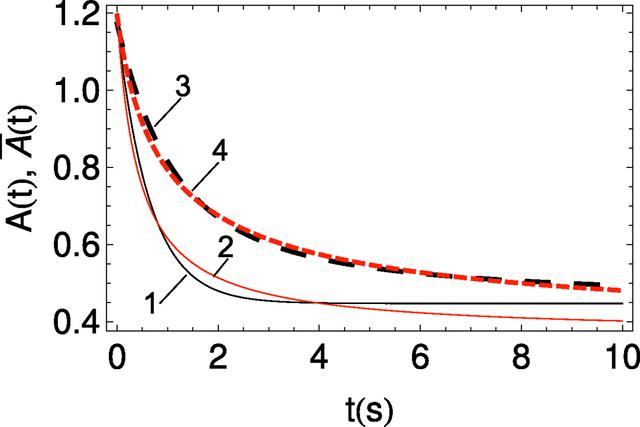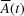disable zoom     view article Figure 10 Comparison of instantaneous A(t) and effective(averaged) optical densities calculated for the Wang method. Black (1, 3) is model 1 (exponential) and red (2, 4) is model 2 (hyperbolic). Solid curves (1, 2) are the instantaneous A(t) – an approximation of the direct STXM data; dashed curves (3, 4) are the effective(averaged) over the time interval t.JOURNAL OFSYNCHROTRONRADIATION
ISSN: 1600-5775
Volume 25| Part 3| May 2018| Pages 833-847